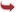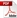International Journal of Scientific and Research Publications

#### IJSRP, Volume 4, Issue 5, May 2014 Edition [ISSN 2250-3153]An Introduction to Tetryonic Theory
Kelvin C. ABRAHAM
Abstract: Tetryonics is a new geometric theory of mass-ENERGY-Matter and the forces of motion, stemming from a geometric re-interpretation of what squared numbers are in physics and its application to quantized angular momentum (QAM). It can now be shown through geometry that the QAM of Planck’s constant is in fact the result of its quantized equilateral ‘triangular’ geometry (and not a vector rotation about a point as has been historically assumed to date).
[VIEW FULL PAPER][DOWNLOAD]

Reference this Research Paper (copy & paste below code):

Kelvin C. ABRAHAM (2018); An Introduction to Tetryonic Theory; Int J Sci Res Publ 4(5) (ISSN: 2250-3153). http://www.ijsrp.org/research-paper-0514.php?rp=P292642